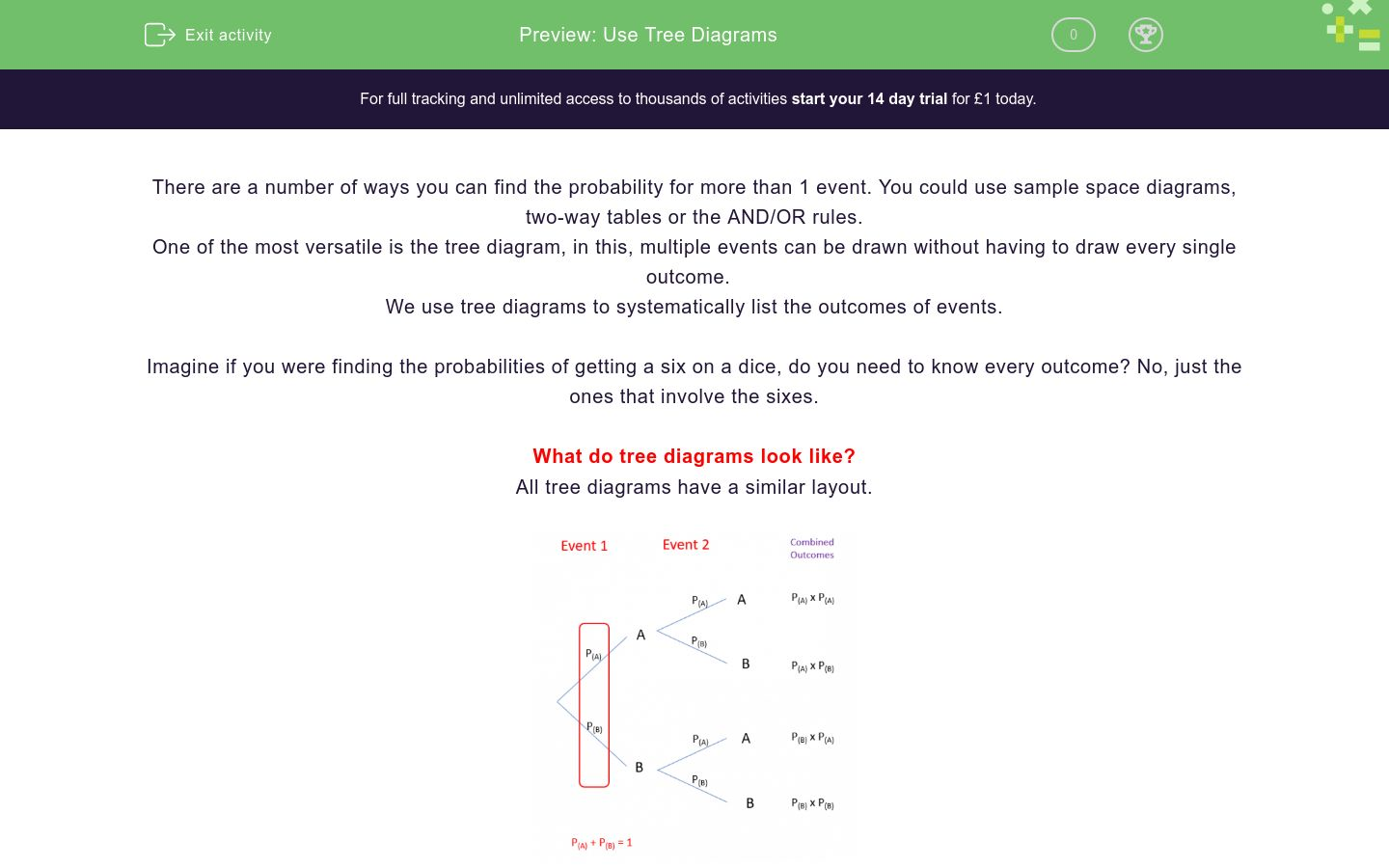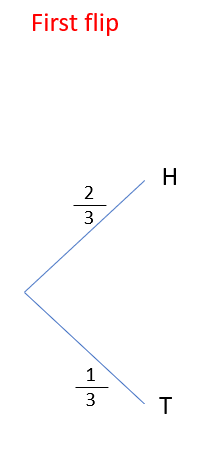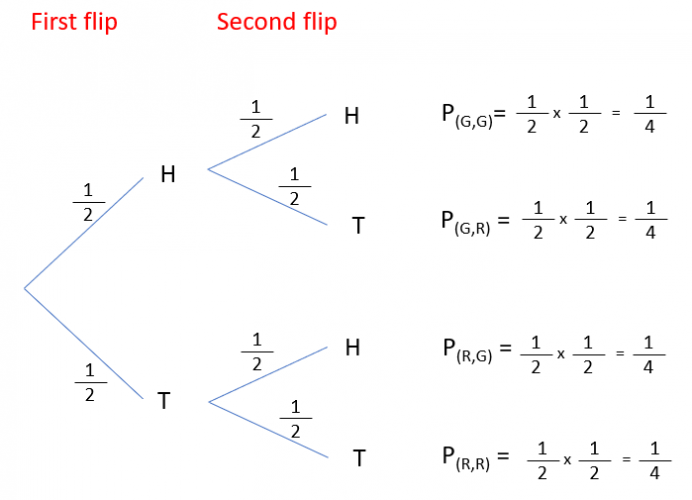# Use Tree Diagrams

In this worksheet, students practise creating and using tree diagramsKey stage:  KS 4

GCSE Subjects:   Maths

GCSE Boards:   AQA, Eduqas, Pearson Edexcel, OCR

Curriculum topic:   Probability

Curriculum subtopic:   Probability, Combined Events and Probability Diagrams

Difficulty level:### QUESTION 1 of 10

There are a number of ways you can find the probability for more than 1 event. You could use sample space diagrams, two way tables or the AND/OR rules.

One of the most versatile is the tree diagram, in this, the outcomes of multiple events can be drawn without having to draw every single outcome.

Imagine if you were finding the probabilities of getting a six on a dice, do you need to know every outcome? No, just the ones that involve the sixes.

What do tree diagrams look like?

All tree diagrams have a similar layout.At first glance, this looks really complicated, but let's work it through with an example.

Example 1: I flip a weighted coin twice where P(H) = 2/3, draw a tree diagram to show all the outcomes.

In this question, we know a couple of things straight away.

1) We know that the chance of getting a head is 2/3, this means the probability of getting tails is 1/3 (These have to add to 1)

2) We are flipping the coin twice. This means we have two events with two outcomes each.

Let's start by looking what could happen the first time we flip the coin,We can get either head or tail, that's it.

Let's now look at the second branches. If I got a head on the first flip, it wouldn't change what I could get or the probabilities so I can draw another branch.I can then also do the same for if I get tails the first time.Now I have the structure, I can start to look at the outcomes, You should be able to see from this tree diagram that if I flip a coin twice, I could get 4 different outcomes H,H  H,T  T,H and TT.I now need to find the probabilities of each of these outcomes.

For example. P (HH) = P(H) x P(H) = 2/3 x 2/3 = 4/9The last step to check we have done this correctly is to look at these 4 new probabilities at the end.They describe everything that could happen, so they must add to 1.

How do we use this?

Tree diagrams are all about finding probabilities for combined events. Lets look at an example.

What is the probability that I throw the coin twice and get one head and one tail?

There are two ways this can happen. HT and TH.

All we have to do is find the ones on the tree that show this (They're the middle 2) and add them together.

2/9 + 2/9 = 4/9

Complete the following sentence.

A normal coin is flipped twice.

For the tree diagram...Find the values of A, B, C and D

{HINT - You will find it easier for this, and the questions that are next, if you draw this out on paper first}

 Probability A B C D

A normal coin is flipped twice, which gives the tree diagram..What is the probability I will get two heads?

1/2

1/4

1

A normal coin is flipped twice, which gives the tree diagram..What is the probability I will get at least one head?

On my drive into work, I go past two sets of lights. The probability that either will be green is 3/4

For the tree diagram...Find the values of A, B, C and D

{HINT - You will find it easier for this, and the questions that are next, if you draw this out on paper first}

 Probability A B C D

On my drive into work, I go past two sets of lights. The probability that either will be green is 3/4What is the probability I will have to stop twice

1/4

1/16

On my drive into work, I go past two sets of lights. The probability that either will be green is 3/4What is the probability I will have to stop exactly once

6 out of every 10 cars in the UK are British, I look at two cars going past.

Complete the tree diagram...Find the values of A, B, C and D

≠B means NOT British

{HINT - You will find it easier for this, and the questions that are next, if you draw this out on paper first}

 Probability A B C D

6 out of every 10 cars in the UK are British, I look at two cars going past.What is the probability the first car will be British and the second will not be British

6/25

12/25

6 out of every 10 cars in the UK are British, I look at two cars going past.What is the probability I will see exactly one British car?

6/25

12/25

• Question 1

Complete the following sentence.

EDDIE SAYS
The whole point of tree diagrams is to systematically list the outcomes (the things that happen) for more than 1 event (the things we do).
• Question 2

A normal coin is flipped twice.

For the tree diagram...Find the values of A, B, C and D

{HINT - You will find it easier for this, and the questions that are next, if you draw this out on paper first}

 Probability A B C D
EDDIE SAYS
This is quite an easy one. The probability for the second branches will all be 1/2. All we then have to do is multiply 1/2 x 1/2 for each branch.
• Question 3

A normal coin is flipped twice, which gives the tree diagram..What is the probability I will get two heads?

1/4
EDDIE SAYS
People often make mistakes here. To find the final outcomes from a tree diagram, we multiply the probabilities of each event. 1/2 is the probability I get heads on a single die 1 is where the pupil has added the probability instead of multiplying (Think that 1 means certain in probability, is it certain I'll get two heads?)
• Question 4

A normal coin is flipped twice, which gives the tree diagram..What is the probability I will get at least one head?

3/4
EDDIE SAYS
You need to start this question by asking how this could happen. There are 3 ways this could happen. (Head Head, Head Tail and Tail Head) All we have to do is add the three probabilities for these events together.
• Question 5

On my drive into work, I go past two sets of lights. The probability that either will be green is 3/4

For the tree diagram...Find the values of A, B, C and D

{HINT - You will find it easier for this, and the questions that are next, if you draw this out on paper first}

 Probability A B C D
EDDIE SAYS
The probability for the second branches will be the same as the first All we need to do is multiply for each branch, so for example, P (G,G) = 3.4 x 3/4 = 9/16
• Question 6

On my drive into work, I go past two sets of lights. The probability that either will be green is 3/4What is the probability I will have to stop twice

1/16
EDDIE SAYS
People often make mistakes here. To find the final outcomes from a tree diagram, we multiply the probabilities of each event. 1/2 is the probability I stop at a single set of lights, not both
• Question 7

On my drive into work, I go past two sets of lights. The probability that either will be green is 3/4What is the probability I will have to stop exactly once

3/8
EDDIE SAYS
You need to start this question by asking how this could happen. There are 2 ways this could happen. (Red Green and Green Red) All we have to do is add the two probabilities for these events together
• Question 8

6 out of every 10 cars in the UK are British, I look at two cars going past.

Complete the tree diagram...Find the values of A, B, C and D

≠B means NOT British

{HINT - You will find it easier for this, and the questions that are next, if you draw this out on paper first}

 Probability A B C D
EDDIE SAYS
Did you work out that the probability of it not being British was 2/5 (Remember that they add up to 1) The probability for the second branches will be the same as the first All we need to do is multiply for each branch, so for example, P (B,≠B) = 3/5 x 2/5 = 6/25
• Question 9

6 out of every 10 cars in the UK are British, I look at two cars going past.What is the probability the first car will be British and the second will not be British

12/25
EDDIE SAYS
This is simply a case of working out which one of the outcomes works. I specified B, ≠ B. In this, the order does matter so the only one that works is the second one down.
• Question 10

6 out of every 10 cars in the UK are British, I look at two cars going past.What is the probability I will see exactly one British car?

12/25
EDDIE SAYS
You need to start this question by asking how this could happen. There are 2 ways this could happen. (B, ≠B or ≠B,B) All we have to do is add the two probabilities for these events together
---- OR ----

Sign up for a £1 trial so you can track and measure your child's progress on this activity.

### What is EdPlace?

We're your National Curriculum aligned online education content provider helping each child succeed in English, maths and science from year 1 to GCSE. With an EdPlace account you’ll be able to track and measure progress, helping each child achieve their best. We build confidence and attainment by personalising each child’s learning at a level that suits them.

Get started